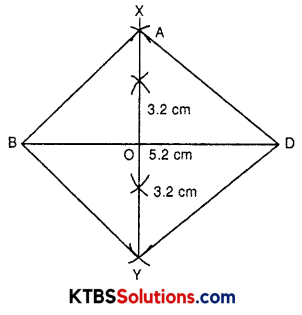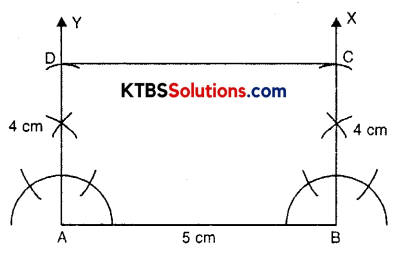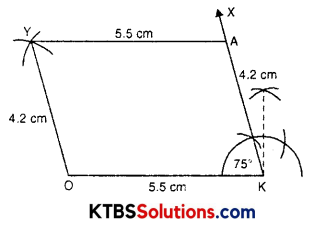# KSEEB Solutions for Class 8 Maths Chapter 4 Practical Geometry Ex 4.5

You can Download KSEEB Solutions for Class 8 Maths Chapter 4 Practical Geometry Ex 4.5 Questions and Answers helps you to revise the complete syllabus.

## KSEEB Solutions for Class 8 Maths Chapter 4 Practical Geometry Ex 4.5

Question 1.
Draw the square READ with RE = 5.1 cm.
Solution:
In a square all sides are equal and all angles are 90° each.
Steps of construction:

1. Draw a line – segment RE = 5.1 cm.
2. Make ∠YRE = 90° and ∠REX = 90°.
3. Cut off DR = AE = 5.1 cm.
5. DEAR is a required square.Question 2.
Draw a rhombus whose diagonals are 5.2 cm.
Solution:
Diagonals of a rhombus bisect perpendicularly.Steps of construction:

1. Draw diagonal BD = 5.2 cm.
2. Draw perpendicular bisector of BD, take it XY which intersect BD at O.
3. Cut off OA = 3.2 cm and OC = 3.2 cm on the opposite side of BD.
4. Join A and C to B and D.
5. ABCD is a required rhombus.Question 3.
Draw a rectangle with adjacent sides of length 5 cm and 4 cm.
Solution:
In a rectangle, each angle is 90° and opposite sides are equal.
Steps of construction:

1. Draw a line segment AB = 5 cm.
2. Construct ∠A = ∠B = 90°.
3. Cut off AD = BC = 4 cm.
4. Join CD.
5. ABCD is a required rectangle.Question 4.
Draw a parallelogram OKAY where OK = 5.5 cm and KA = 4.2 cm.
Solution:
In a parallelogram opposite sides are equal.
Steps of construction:

1. Draw a line segment OK = 5.5 cm.
2. Take ∠K = 75° and construct it, such that ∠OKX = 75°.
3. Cut off KA = 4.2.
4. From A draw an arc of radius 5.5 cm.
5. From O draw an arc of radius 4.2 cm.
6. Both these arcs intersect at Y.
7. Join OY and AY.
8. OKAY is a required parallelogram.